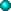Updated RegularlyClick REFRESH on your browser if pages seem unchanged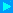Visit our amazing document server. Everything from Quotes and SciFi to Business and Ethics.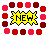CLICK for WHAT's NEW Sphere Research Corporation3394 Sunnyside Rd.West Kelowna, BC, Canada V1Z 2V4Phone: +1 (250) 769-1834FAX:+1 (250) 769-4106 A great source for test equipment, repairs, calibrations, useful metrology information, and of course, SLIDE RULES!JUST SCROLL DOWN TO SEE EVERYTHING!How and Why a Slide Rule Works!Original Artwork by: Larry Stewart, The Slide Rule Universe is a trademark of Sphere Research Corporation

Article by: John Savard, copyright 2001
Reproduced by permission of the author.

The diagram below illustrates the appearance of that most mysterious artifact of antiquity, the slide rule.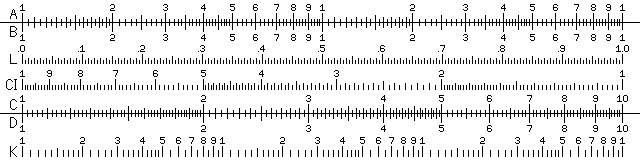How could such a strange-looking object be used to perform calculations?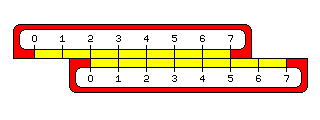The underlying principle of the slide rule is simple enough. If you take two ordinary rulers, and starting from a position of two units - two inches, two centimeters, whatever you care to imagine - measure a further three units with another ruler, you will obtain a distance of five units - which the first ruler will indicate.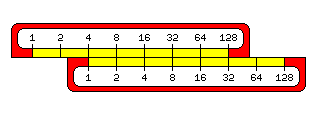Multiplication behaves like addition. If you multiply 2 by itself over and over, you will get the powers of two, and 8 times 32 is the same as 16 times 16, just as 3 plus 5 is the same as 4 plus 4. If, instead, you multiplied a very small number by itself, you could find at least an approximate place to put every number in order to multiply by adding. There are, however, easier ways to calculate logarithms. The logarithm function is the inverse of the power function. Thus, 4 is 2 to the second power, 8 is 2 to the third power, 16 is 2 to the fourth power, and 2 is the logarithm of 4 to the base 2, 3 is the logarithm of 8 to the base 2, and 4 is the logarithm of 16 to the base 2. Common logarithms are logarithms to the base 10. This makes them convenient for doing arithmetic, because the integer part of a common logarithm indicates where to put the decimal point, and the fractional part is the same for the logarithm of 326, 32.6, or 3.26, so that tables need only contain the fractional part of a common logarithm. Natural logarithms are logarithms to the base e, where e is 2.71828..., a fundamental constant like pi. These logarithms are more fundamental when working with calculus, but aren't as useful for everyday calculations. But while common logarithms are the basis of the slide rule, natural logarithms will turn up when we examine more advanced scales.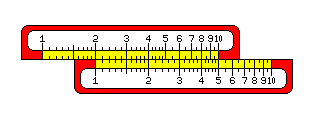Here we see two slide rule scales set up to multiply the numbers on the bottom scale by 2. Thus, 1 was placed under the 2; therefore, 2 is under the 4, 3 is under the 6, 4 is under the 8, and 5 is under the 10.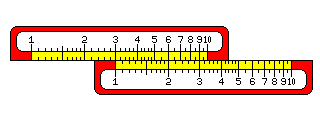This works just as well for other numbers; here, the 1 is placed under the 3, and so the 2 is under the 6, and the 3 is under the 9.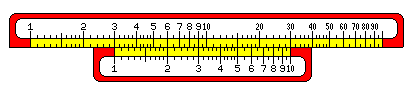But what happens to the other numbers when they're multiplied by 3? One way to find out would be to add a second scale to the end of the top scale. This way, we see the following: The small tick marks between "10" and "20" divide the area between them into ten parts, so each small tick mark in that area stands for 1. Thus, we can see that the 4 stands under 10 and two tick marks, or 12. Similarly, 5 stands under 15, and 6 stands under 18. From 20 to 50, there are only five tick marks between each number and the number that is 10 greater. So each tick mark stands for 2. Thus, we see that 3 times 7 is 21, since the 7 is under a point about halfway between the 20 and the first tick mark following it. On the other hand, that 3 times 8 is 24 is easy to see, as that falls under the second tick mark after 20. And, since 3 times 9 is 27, again we see that the 9 is between the tick marks that represent 26 and 28. Finally, the 10 falls under the 30.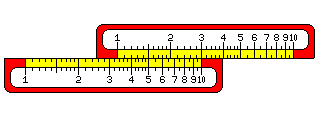Noting how the scales on a slide rule simply repeat themselves, one can put the 10 instead of the 1 under the number by which one wishes to multiply to see the other possible products. This lets one use the C and D scales, instead of the A and B scales, which are on a smaller scale, to multiply, giving more accuracy.

Having seen how to do basic multiplication on a slide rule, we can now understand the basic scales on a slide rule, as shown in the first image above.

If you multiply a number by itself, the result will be twice the distance from the index - the 1 mark - as the original number was. So if you have two slide rule scales, one twice as big as the other, if their indexes are aligned, each number on the scale that is twice as big corrsponds to the location of its square on the smaller scale.

Thus, the A and B scales, at half the side, with the scales repeated twice, aren't just for beginning slide rule users. Rather, they let people work with squares and square roots.

Similarly, the K scale is at one third of the scale of the C and D scales, and so it is used for cubes and cube roots.

The CI scale is the same as the C scale, except it runs backwards. Since division is the opposite of multiplication, this scale can be used to find reciprocals.

Of course, it's obvious from the way that multiplication is performed on a slide rule that you can perform division without the need for such a scale; going from 3 to 1 measures out the same distance backwards as going from 1 to 3 does forwards. So if you place the 1 on one scale under the 3 on the other, not only can you see 3 times any number on the bottom scale on the top scale, but you can also see any number on the top scale divided by 3 on the bottom scale.

The L scale is a uniform linear scale, and is used to read off the fractional portion (or mantissa) of the common logarithm of numbers on the C scale.

This explains the simple scales on slide rules. Many slide rules, however, have more complicated scales on them as well.

The S scale has marks that are labelled with angles in degrees; the location of each mark corresponds to the position on the C scale where the sine of that angle is found. Similarly, the T scale has marks that are labelled with angles in degrees, and the location of each mark corresponds to the position on the C scale where the tangent of that angle is found.

Thus, the S and T scales can be used for multiplying a number by the sine or tangent of an angle.

The tangent of an angle over 45 degrees is the reciprocal of the tangent of 90 degrees minus that angle. Thus, one uses the T scale to divide instead of multiply for such large angles. The number of degrees for such angles is thus noted in red on most slide rules, in the same way that the numbers on the CI scale usually are.

Usually, the center slide of even a simple slide rule had S and T scales; normally, you flipped the center part over by taking it out of the side of the slide rule first, then flipping it over and putting it back in. The L scale was usually between the S and T scales on the back side of the center slide.

The fancier slide rules also had a set of scales called "log log scales". What these scales let you do was raise numbers to arbitrary powers.

If the K scale, for example, shows the cube of numbers on the D scale by including three scales in the space of one, because adding the logarithm of a number to itself three times produces the logarithm of its cube, then one can cube a number by multiplying its logarithm by three.

And in that case, since a slide rule performs multiplication, a suitable scale should allow exponentiation.

This diagram illustrates a slide rule with a very small-scale log-log scale, matching the K scale. Usually, the log-log scale shown here would instead be broken into three pieces, called LL1, LL2, and LL3, matching the C scale. The coarse resolution of the diagram above, and its small scale, shows the mark for 1.001 on the LL scale coinciding with the 1 on the K scale, but when the scale is made to correspond with the C scale, on a real 10 inch slide rule, a fourth piece, extending from 1.0001 to 1.001, called the LL0 scale, is worthwhile to provide, even if, for smaller numbers, the D scale will serve.

By shrinking the log-log scale down, I paired a scale with both it and the K scale in the diagram, to make the illustration simpler to understand.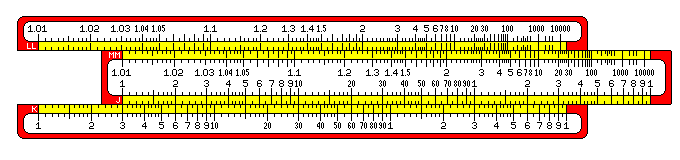The J scale has been moved so that the 1 on that scale corresponds to the 3 on the K scale. Thus, every number on the J scale is multiplied by three on the K scale.

This displacement means that every number on the MM scale is cubed on the LL scale.

Thus, 1.01 is just past 1.03; 2 is under 8, 3 is under 27, 10 is under 1000.

Notice that the distance between numbers quickly shrinks. Thus, the tick marks between 100 and 1000 represent 150, 200, 250, 300, 350, 400, and 500.

While 10, 100, and 1000 stand in the same relation to each other on the LL scale as 1, 2, and 3 do on the K scale, note that 1.01, 1.02, and 1.03 also stand in approximately the same relationship to each other as 1, 2, and 3 do on the K scale.

This would let the K scale itself stand in for a lower extension of the LL scale, dealing with numbers like 1.001, 1.0001, and 1.00001. So the LL scale is aligned to facilitate that; 1.01 is placed near the index, instead of 10 being placed directly over 100 on the K scale.

This is done by placing a number on the log-log scale as follows: first, its natural logarithm is taken (just as the sine is taken first for the S scale), and then the common logarithm of that is taken to fit in with the other scales for multiplication. 1.01 to the 100th power is very nearly equal to e, and 1.001 to the 1000th power is even closer, and so on. So this is where we meet natural logarithms on a slide rule.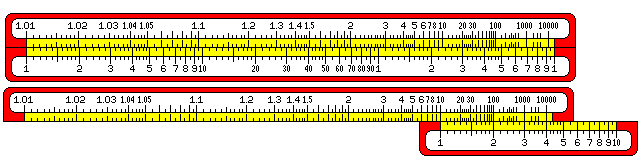This diagram, placing the K scale directly against the LL scale, illustrates this more clearly, showing the two cases, the first where the correspondence shows with an alignment based on natural logarithms, and is an approximation approached in the limit, and the second where the correspondence is exact, and shows with an alignment based on common logarithms.

Incidentally, while most slide rules with log-log scales aligned them based on natural logarithms, one maker (Pickett) did use the common logarithm alignment. Also, some very old slide rules did have a log-log scale which was matched to the K scale for simplicity.

Many, but not all, slide rules with log-log scales also had reciprocal log-log scales (LL/3, LL/2, LL/1, LL/0 or LL03, LL02, LL01 and LL00) with numbers on them that were the reciprocals of those on the corresponding log-log scale, to allow numbers between 0 and 1 to be raised to powers as well without taking an extra step to calculate their reciprocals.

Most slide rules with both log-log and reciprocal log-log scales put the LL0 and LL/0 scales on the other side of the slide rule from the rest of the log-log scales; this was because all the ordinary scales were on the front, but there wasn't quite enough room for both log-log scales in full on the back. One of the few slide rules that avoided this was the Deci-Lon slide rule from Keufel and Esser, which is today one of the slide rules most sought after by collectors. It placed the four log-log scales on one side, and the four reciprocal log-log scales on the other. The Faber-Castell Novo Duplex, however, did even better, having all four segments of both log-log scales on one side. It was also unique in having two sets of double-length scales for square roots which slid against each other, allowing higher accuracy in multiplication.

Also, some slide rules had other special scales. These are a few of the more common:

• CF: This was a "folded" C scale, which was simply shifted so that the numbers marked on it were pi times the number on the C scale.
• CIF: This scale showed the reciprocals of the numbers on the CF scale.
• R1, R2 (or W1, W2): Instead of A and B scales, or in addition to them, some slide rules had a scale twice as long as the C scale, split into two halves to fit on the slide rule. This allowed calculations of square roots to be done with greater accuracy. (This was done on Versalog slide rules, for example.)
• T1, T2: Some slide rules included a second T scale so that tangents of angles over 45 degrees could be directly represented, instead of using additional (usually red) numbers indicating 90 degrees minus the angle on the T scale to allow the cotangent (the reciprocal of the tangent) of those angles to be found.
• ST or SRT: This is a folded C scale. Finding an angle on this scale in degrees allowed its equivalent in radians to be read off on the C scale. As sin(x) and tan(x) are very nearly x in radians for very small x, the primary use of this scale was to provide a backwards extension to the S and T scales.
• P: A number of slide rules, particularly those from Aristo, had this, the "Pythagorean" scale. A number x on this scale corresponded to sqrt( 1 - x^2 ) on the C scale (and vice versa, as this function is self-inverse) where the C scale is considered as containing the numbers from .1 to 1. This function was also one of the circle functions in APL along with the trigonometric functions, and could be used in calculating one side of a right triangle from the two others. This scale rapidly becomes very compressed near the right-hand side of the slide rule, so the fact that the function is self-inverse is helpful when using it for looking up the value instead of for multiplication in a subsequent step.
• Ln: A linear scale on a smaller scale than the L scale, this gave the natural logarithm of numbers from 1 to 10. Note that the log-log scales give natural logarithms in the same fashion that the S and T scales give sine and tangent, looking up the number on the scale which is the argument of the function, and finding the function's value on the C or D scale. This is the usual way in which scales associated with a function work, as this allows multiplying by that function of a number. But one doesn't usually multiply by a logarithm, and laying out the L and Ln scales in the reverse manner allows these functions to be looked up with constant percentage error in the argument and constant error in the result, which is a more reasonable distribution of error for logarithms.
• BI: This scale was like the B scale, except that it ran backwards, bearing the same relation to the B scale as the CI scale did to the C scale. Thus, it allowed one to more quicly multiply by the reciprocal of a square root, by bringing the index of the slide against a number on the D scale instead of using the cursor.
• 2 pi: Electronic slide rules sometimes had a folded C scale which was folded at 1/(2*pi) instead of at pi. This was useful for calculations of the reactance of a capacitor (1/(2*pi*f*C)) or an inductor (2*pi*f*L).
• H or LC: This scale was like a BI scale, but was folded at 1/((2*pi)^2), which is approximately 0.02533. The resonant frequency of a tuned circuit with inductance L and capacitance C is 1/(2*pi*sqrt(L*C)), so one could divide either L or C on this scale by the other one on the B scale to read the resonant frequency on the D scale.
• Th, Sh 1, Sh 2: Also, some slide rules had scales for some of the hyperbolic functions, hyperbolic tangent and hyperbolic sine. The hyperbolic sine occupied a split double-length scale.
• Sd, Td, ISd, ITd: These scales show the ratio of the sine, tangent, inverse sine, and inverse tangent to their arguments (in degrees). These four scales have ranges that do not overlap, and thus they only occupy the same space as a single normal full-length scale. While these ratios are not often used directly in calculations, these scales can be used to provide sines and tangents with the same accuracy, and over the same range, as the complete set of four full-length scales S1, S2, T, and ST, and thus one maker of slide rules (P.I.C.) used these scales as a replacement for conventional trigonometric scales on some models of slide rule, despite the fact that this means that multiplying a quantity by the sine of an angle would take two steps instead of one.

Slide rules with the scales for calculating reactance and resonant frequency often also had smaller versions of those scales on the back (repeated, and labelled with explicit magnitudes) to assist in locating the decimal point in such calculations.

Slide rules for business use sometimes had a linear scale which was used for calculating the number of days between two dates. A similar scale could be used to make a perpetual calendar: the days of the week might be symbolized by a pattern of tick marks like this:

``` ||||||||||||||
|||||  |||||
|||||  |||||
| | |  | | |
```

with short ticks representing the weekend, and the longest ticks representing Monday, Wednesday, and Friday so that the alternating pattern allows recognizing any day of the week quickly. The scale of days of the week could be positioned against the scale showing the days of the year by bringing, further along the scale, a mark for the century opposite a mark for the year of the century.

Note that the illustration of a simple slide rule at the top of the page corresponds to the distribution of tick marks on a 5-inch slide rule (actually, one with a 12.5 cm scale), not the usual 10-inch (25 cm) slide rule. Despite this, and despite the use of 600 pixels to represent the scale width, the positions of the tick marks are noticeably uneven. Because slide rules have scales which gradually vary in size, they are very difficult to depict well in discrete pixels.

A BASIC program was used to draw a picture in the .xbm file format, containing the plain scales with ticks of varying size and spacing but no numerals, which I then viewed with a web browser, and got into a paint program by using the clipboard.

A width of 600 pixels was chosen to allow the L scale, at least, to appear uniform, and to allow the two repeated occurences of the scale on the A and B scales, and the three occurences on the K scale, to be identical.

For the other diagrams on this page, one copy of the scale from the K scale was used to represent the C and D scales, and a slightly smaller scale was used, so that the distance between 1 and 2 was exactly 53 pixels, and the distance between 1 and 10 was 176 pixels. (The same program drew the log-log scale used in the diagrams above; instead of replying 600 to the prompt for the width of the slide rule, I replied 528, which is three times 176, to obtain the raw materials for those diagrams.) Thus, while the irregularity in the spacing of the ticks could not be eliminated, at least it repeated at convenient intervals so that the rules could be shown sliding against each other in diagrams which gave a reasonable appearance without having to cheat by shifting some of the ticks by hand from their calculated positions. (I had tried other possibilities, involving approximations only to log(2), but because that is 0.3010299... rational approximations to it, while good, are widely spaced, or involving computer searches for ratios that minimize error over all the tick marks, but they were not as successful.)

Incidentally, some older slide rules have an appearance like this: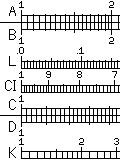Although this makes them more difficult to read, by isolating the eye from the varying heights of the ticks, this arrangement may have been presumed to have the effect of reducing bias in estimating positions between tick marks.

My explanatory diagrams above don't quite use this arrangement; instead, I was trying to dramatize the difference between tick marks further to make the diagrams easier to follow; thus, only major tick marks close a box in what I have drawn, quite the opposite of the older slide rule design.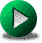Click to visit!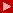Buy a slide rule from our extensive on line marketplace, with restored classics, amazing new in the box rules, and low cost first student rules. We have something for everybody, even a swap and sell area for visitors.Click to visit!Learn to operate and care for your slide rule with these on line manuals and directions. Learn what scales mean, how to use them and how to restore or keep your rule in good condition.Click to visit!Tour our extensive archives of slide rule manufacturers and models from all over the world. Find out who made what, and what became of them after they stopped making slide rules. See how slide rules survive today in the form of slide charts still made in the millions annually.Click to visit!Nice things people have said about us in print and on TV, see the original articles. We always appreciate it!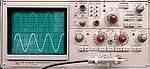Click to visit!Visit our TEST EQUIPMENT site everything from Scopes, CRT's and DMM's to nixie tubes, HP and Tektronix spare parts. A little electronic something for everybody.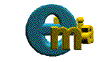E-Mail Us !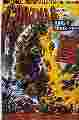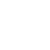You are visitor number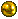. Site Design & contents copyright 1997, 1998, 1999, 2000, 2001, 2002, 2003, 2008, 2013 by Walter Shawlee 2 & the ad hoc Godzilla Graphics Group. Article copyright 2001 by John Savard, reproduced by permission.